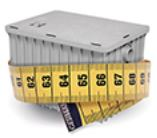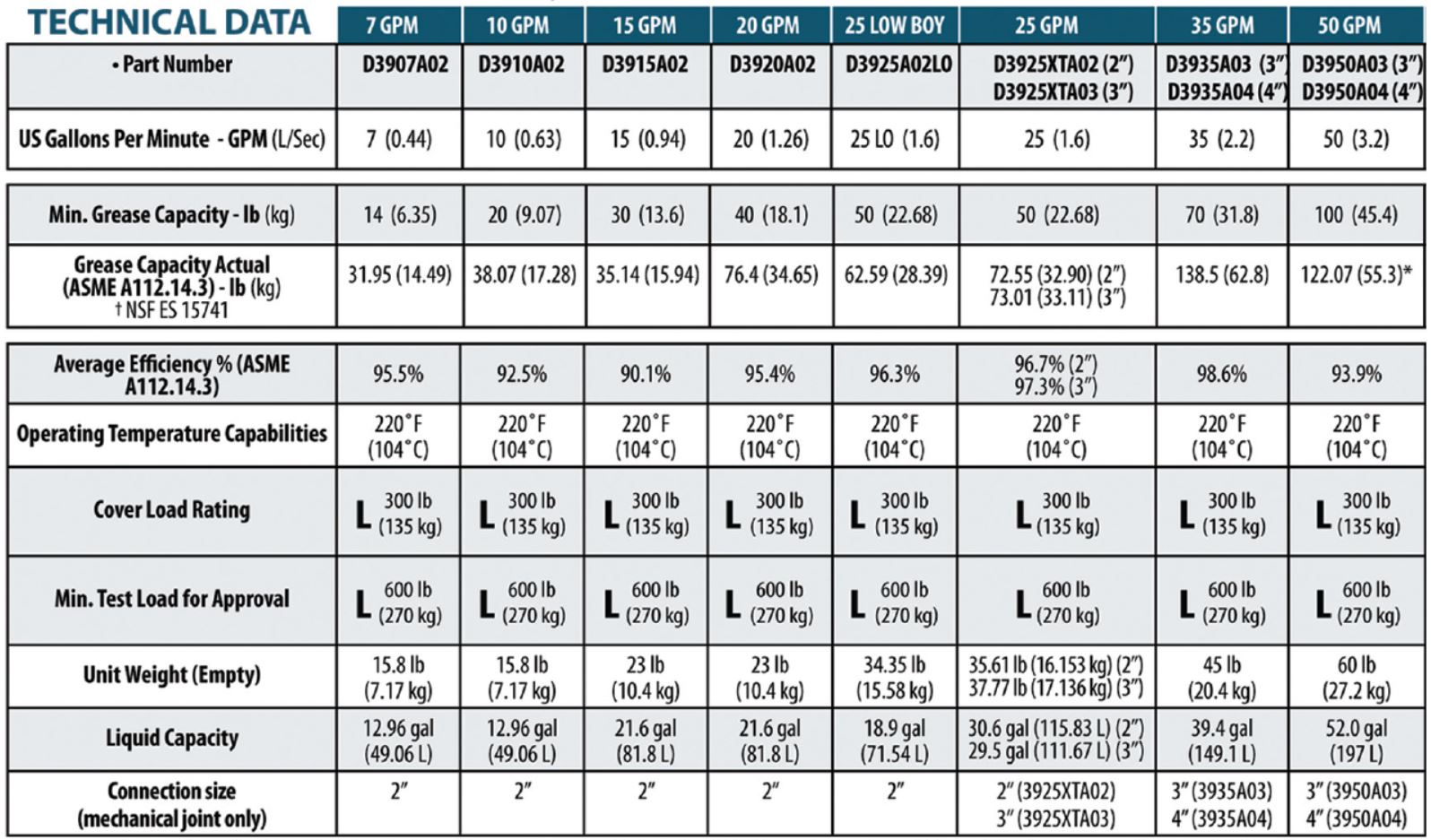# How to size your Grease TrapSizing a Grease Trap by Flow Rate:

Explanatory Note: This method uses total combined fixture capacity to define an actual (maximum) drainage load, which is in turn used to define the flow rating of the interceptor required for the application typically up to 100 GPM.

1. Measure your sinks. Calculate the capacity of the sink in cubic inches by multiplying length by width by depth. If you have multiple compartments or sinks, add the capacity of sinks together to find total cubic inches:
_____ x _____ x _____ = _______ Cubic Inches
Length x Width x Depth

2. Convert the capacity from total cubic inches to gallons per minute (GPM) by dividing by 231:
_______ Cubic Inches ÷ 231 = _______ GPM

3 . Adjust for displacement (displacement takes into consideration the actual usable capacity of your sink):
_______ GPM x 0.75 = _______ GPM Actual Drainage Load for sinks

Note:  Dishwashers can be connected to a Grease Trap only if allowed by local code.  Each dishwasher will require a separate Grease Trap.  For floor drains and floor sinks take the volume of water produced by the number of hose bibs (i.e. 1.5 - 2.0 GPM per 3/4 inch faucet).
____________ + ___________
Actual Drainage Load for sinks + Other Appliances and sinks

5. Determine the flow rate and drainage period. Assume a 1 minute drainage period unless conditions permit a 2 minute drainage period. Drainage period is the actual time it takes to completely drain the fixture.
_______ GPM ÷ ________ drainage period = _________ GPM Total Actual Drainage Load
(1 or 2 min) (Flow Rate)

6. Select interceptor from the table below which corresponds with the flow rate calculated. Round up to the next largest size.  Click here to view and purchase all our Grease Traps

##Example:

This is how you would calculated the size grease trap needed for a typical 3 compartment sink with no additional appliances or other sinks added:
18" x 18" x 10" x 3 (bowls/compartments) = 9720 cubic inches
9720/231 = 42.08 USG
42.08 x 0.75 = 31.56 USG
31.56 / 1 min (or 2 min as applicable) = 31.56 GPM
Next standard size of Grease Interceptor you should use is 35 GPM